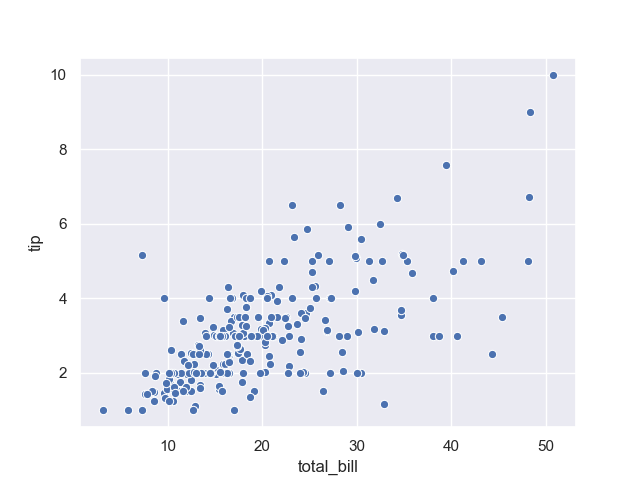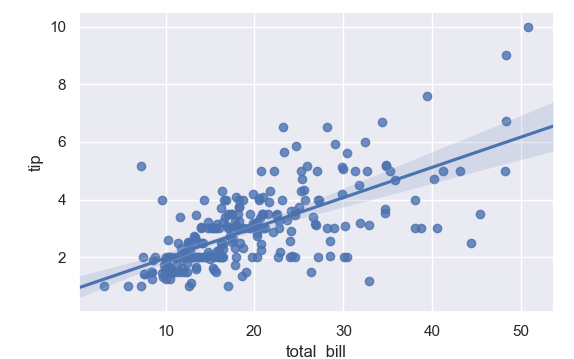The scatterplot is a plot with many data points. It is one of the many plots seaborn can create. Seaborn is a Python module for statistical data visualization.

Seaborn can create this plot with the scatterplot() method. The data points are passed with the parameter data. The parameters x and y are the labels of the plot.

Related course: Matplotlib Examples and Video Course

## Scatterplot

### scatterplot basic

The scatterplot basic plot uses the tips dataset. This is a dataset about tips received based on the total bill. You can use any dataset you want for a scatterplot, this just serves as basic example.### scatterplot

The scatterplot plot can also contain a line. To do that, use an lmplot instead. This nicely shows the relation between the datapoints.